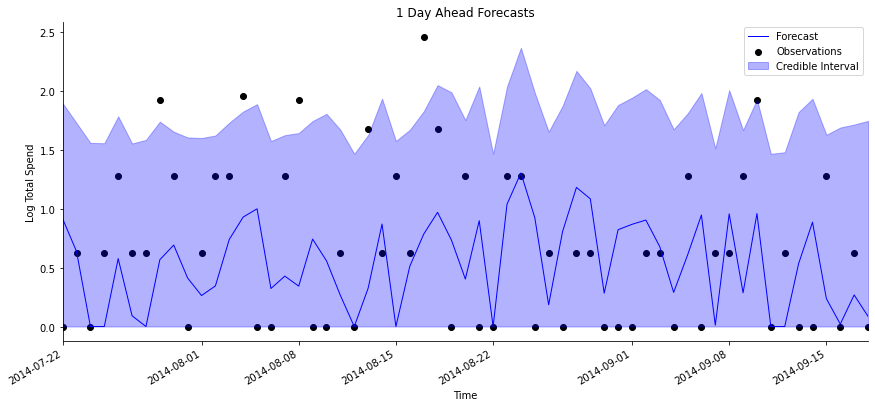A Dynamic Linear Mixture Model, or DLMM, is the combination of a Bernoulli DGLM and a Normal DLM as described in Yanchenko, Deng, Li, Cron and West (2021).

The DLMM was contributed to Pybats in part by Anna Yanchenko.

The DLMM is a combination of a Bernoulli DGLM and a Normal DLM and is motivated by and similar to the dcmm. The Bernoulli DGLM models the probability of the observation being zero. Conditional on a non-zero outcome, then the continuous observation follows a Normal distribution. This is useful for modeling continuous-valued time series with some observations that are exactly zero; under a Normal distribution, the probability of any one observation being identically zero is 0. For example, DLMMs can be used to model series of log total spend, where at some time points, the total spend is identically $0$.

Formally, a DLMM models observations $y_t$ as: $$\quad z_{t} \sim Bern(\pi_{t}) \quad \textrm{and}\quad y_{t} | z_{t} = \begin{cases} 0, & \text{if } z_{t} = 0,\\ x_{t}, \quad x_{t} \sim \mathcal{N}(\mathbf{F}_t'\boldsymbol{\theta}_t, \mathbf{V}_t), & \textrm{if}\ z_{t} = 1. \end{cases}$$

$\boldsymbol{\theta}_t$ follows a DLM evolution involving known regression vectors $\mathbf{F}_t$.

## classdlmm[source]

dlmm(a0_bern=None, R0_bern=None, nregn_bern=0, ntrend_bern=0, nhol_bern=0, nlf_bern=0, seasPeriods_bern=[], seasHarmComponents_bern=[], deltrend_bern=1, delregn_bern=1, delhol_bern=1, delseas_bern=1, dellf_bern=1, rho=1, a0_dlm=None, R0_dlm=None, n0_dlm=1, s0_dlm=1, nregn_dlm=0, ntrend_dlm=0, nhol_dlm=0, nlf_dlm=0, seasPeriods_dlm=[], seasHarmComponents_dlm=[], deltrend_dlm=1, delregn_dlm=1, delhol_dlm=1, delseas_dlm=1, delVar_dlm=1, dellf_dlm=1, interpolate=True, adapt_discount=False)

A DLMM can be used in the same way as a DGLM, with the standard methods dlmm.update, dlmm.forecast_marginal, and dlmm.forecast_path. There are equivalent helper functions as well. A full analysis can be run with analysis_dlmm, and define_dlmm helps to initialize a DLMM. These helper functions assume that the same predictors X are used for the Bernoulli DGLM and the Normal DLM.

The only difference from using a standard dglm is that outside of analysis_dlmm, the update and forecast functions do not automatically recognize whether the DLMM calls a copula for path forecasting. This means that the modeler needs to be more explicit in calling the correct method, such as dlmm.forecast_path_copula for path forecasting with a copula.

A quick example of using analysis_dlmm to model simulated sales data follows.

import pandas as pd
import numpy as np
import matplotlib.pyplot as plt

from pybats.analysis import analysis_dlmm
from pybats.plot import plot_data_forecast
from pybats.point_forecast import median


DISCOUNT_PERC LOG_TOTAL_SPEND
DATE
2014-06-01 0.49 0.62
2014-06-02 0.31 1.28
2014-06-03 0.43 0.00
2014-06-04 0.55 1.28
2014-06-05 0.43 0.00
prior_length = 25   # Number of days of data used to set prior
k = 1               # Forecast horizon
rho = 0.9           # Random effect discount factor to increase variance of forecast distribution
delVar_dlm = 0.95   # Discount factor on the observation variance in the normal DLM
forecast_samps = 1000  # Number of forecast samples to draw
forecast_start = pd.to_datetime('2014-07-21') # Date to start forecasting
forecast_end = pd.to_datetime('2014-09-17')   # Date to stop forecasting

mod, samples = analysis_dlmm(Y = data['LOG_TOTAL_SPEND'].values,
X = data['DISCOUNT_PERC'].values.reshape(-1,1),
prior_length = prior_length,
k = k,
forecast_start = forecast_start,
forecast_end = forecast_end,
nsamps=forecast_samps,
dates=data.index,
rho=rho,
delVar_dlm = delVar_dlm,
ret = ['model', 'forecast'])

beginning forecasting


Because the DLMM is effectively a container for a Bernoulli DGLM and a Normal DLM, we can access each of them individually. The coefficients in the Bernoulli DGLM affect the probability of a non-zero observation, and the coefficients in the Normal DLM impact the value of any non-zero observations.

The $1-$step ahead forecasts are shown below, using the plot_data_forecast function.

forecast = median(samples)

# Plot the 1-day ahead forecast
h = 1
start = np.where(data.index == forecast_start) + h
end = np.where(data.index == forecast_end) + h + 1

fig, ax = plt.subplots(figsize=(12, 6))
plot_data_forecast(fig, ax, y = data[start:end].LOG_TOTAL_SPEND.values,
f = forecast,
samples = samples[:,:,h-1],
dates = data.index[start:end],
xlabel='Time', ylabel='Log Total Spend',